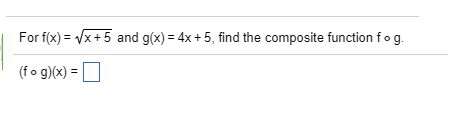1
0
watching
110
views6 Nov 2019

#For f(x)=-/x + 5 and g(x) = 4x + 5, find the composite function fo g (fo g)(x) = ? Show transcribed image text For f(x)=-/x + 5 and g(x) = 4x + 5, find the composite function fo g (fo g)(x) = ?